# RS Aggarwal Solutions for Class 9 Chapter 2: Polynomials Exercise 2D

## RS Aggarwal Solutions for Class 9 Maths Exercise 2D PDF

This is the fourth exercise of the second chapter which contains solutions prepared by subject experts at BYJU’S. Mathematics is one of the major scoring subjects which the students need to assign more importance to and practice accordingly. Some of the topics in class 9 will even be repeated in class 10 so the understanding of fundamental concepts by students is the main aim under this. Exercise 2D of the second chapter has solutions to the problems based on the Factor Theorem. RS Aggarwal Solutions for Class 9 Maths Chapter 2 Polynomials Exercise 2D are provided here.

## RS Aggarwal Solutions for Class 9 Chapter 2: Polynomials Exercise 2D Download PDF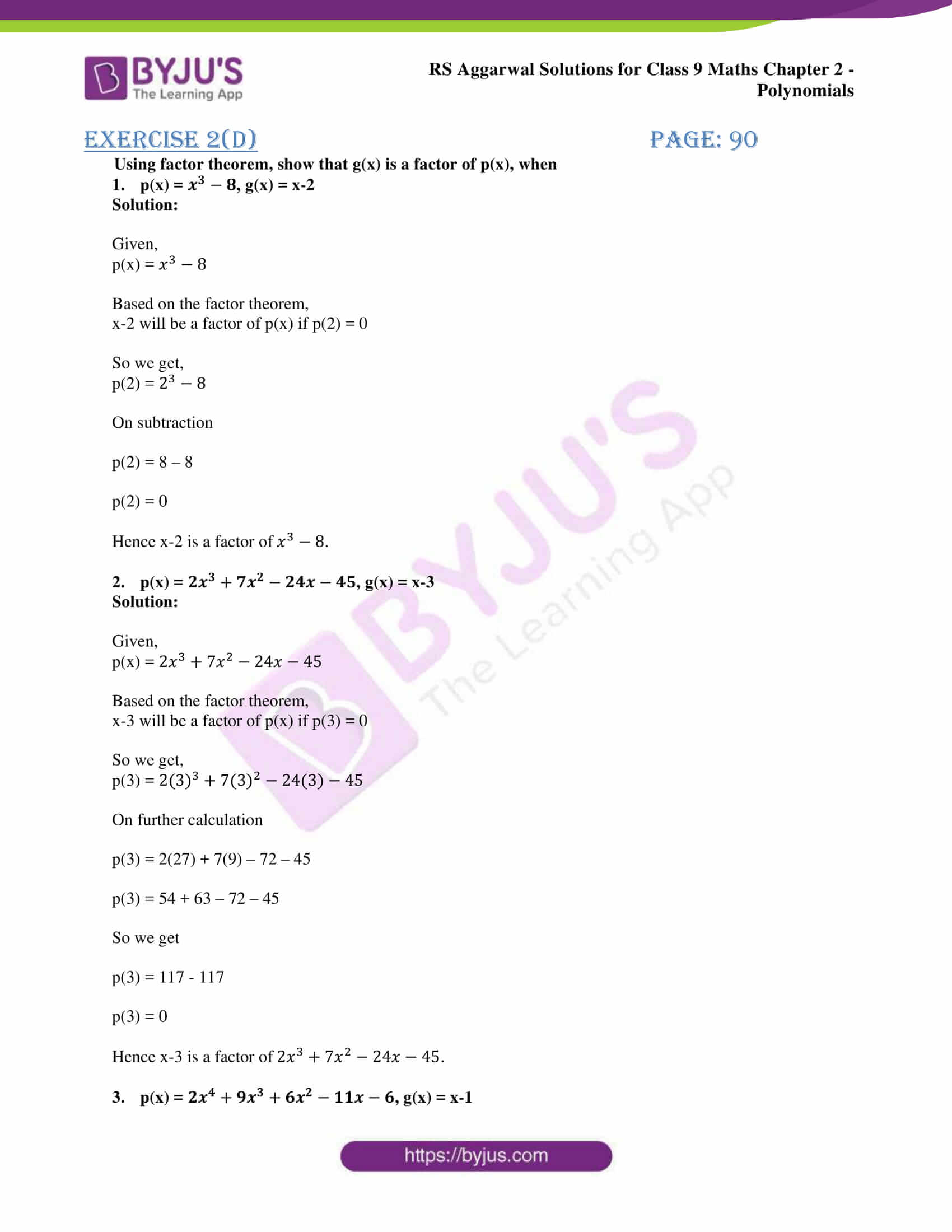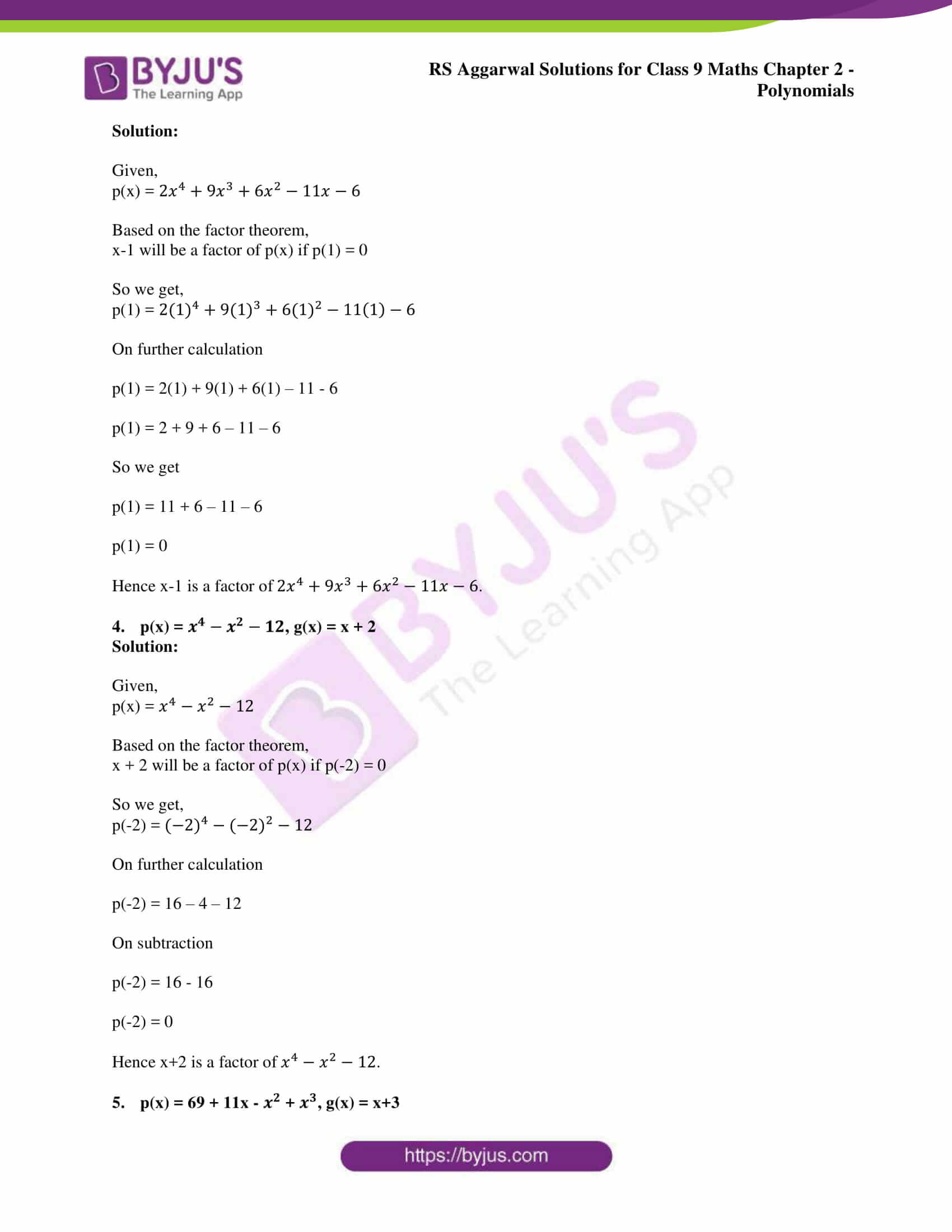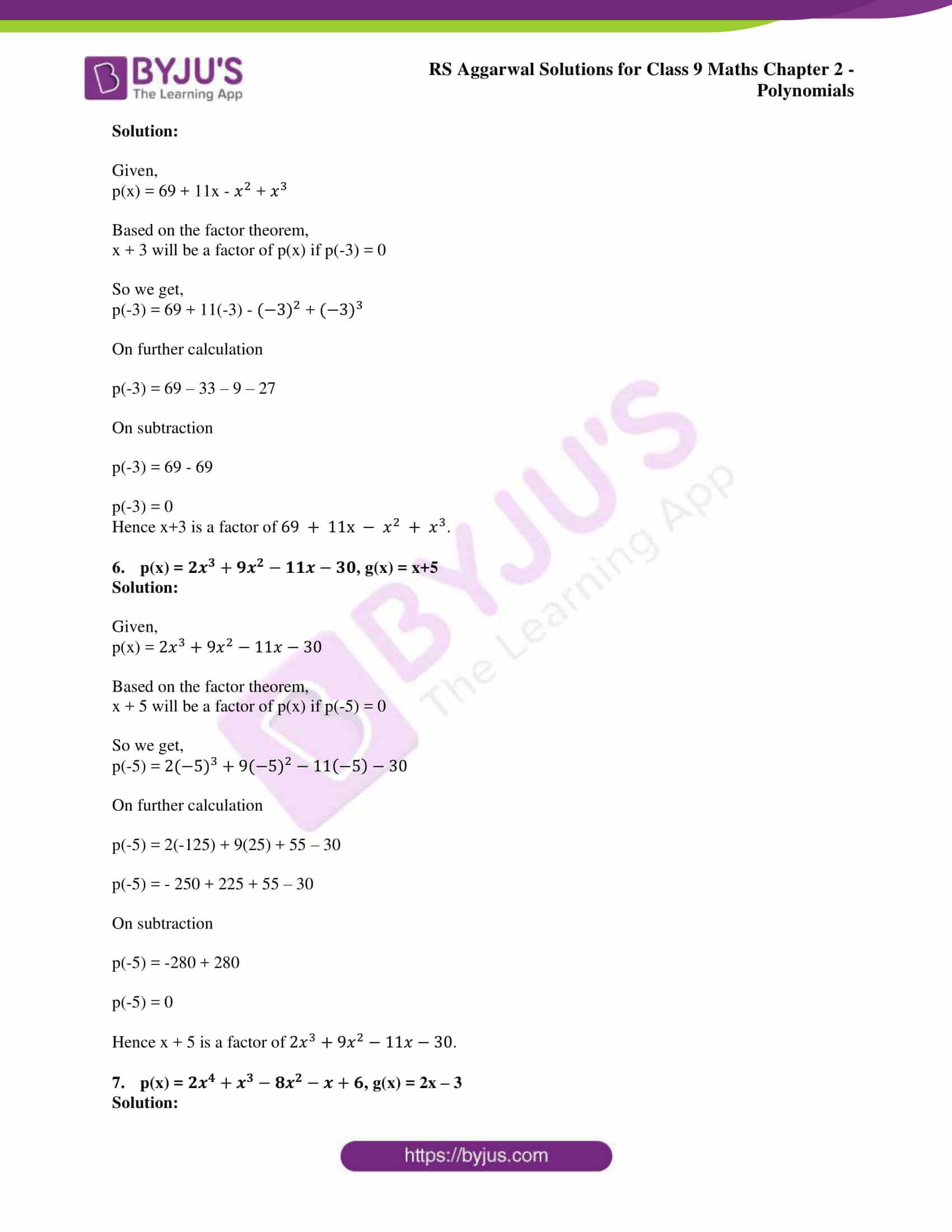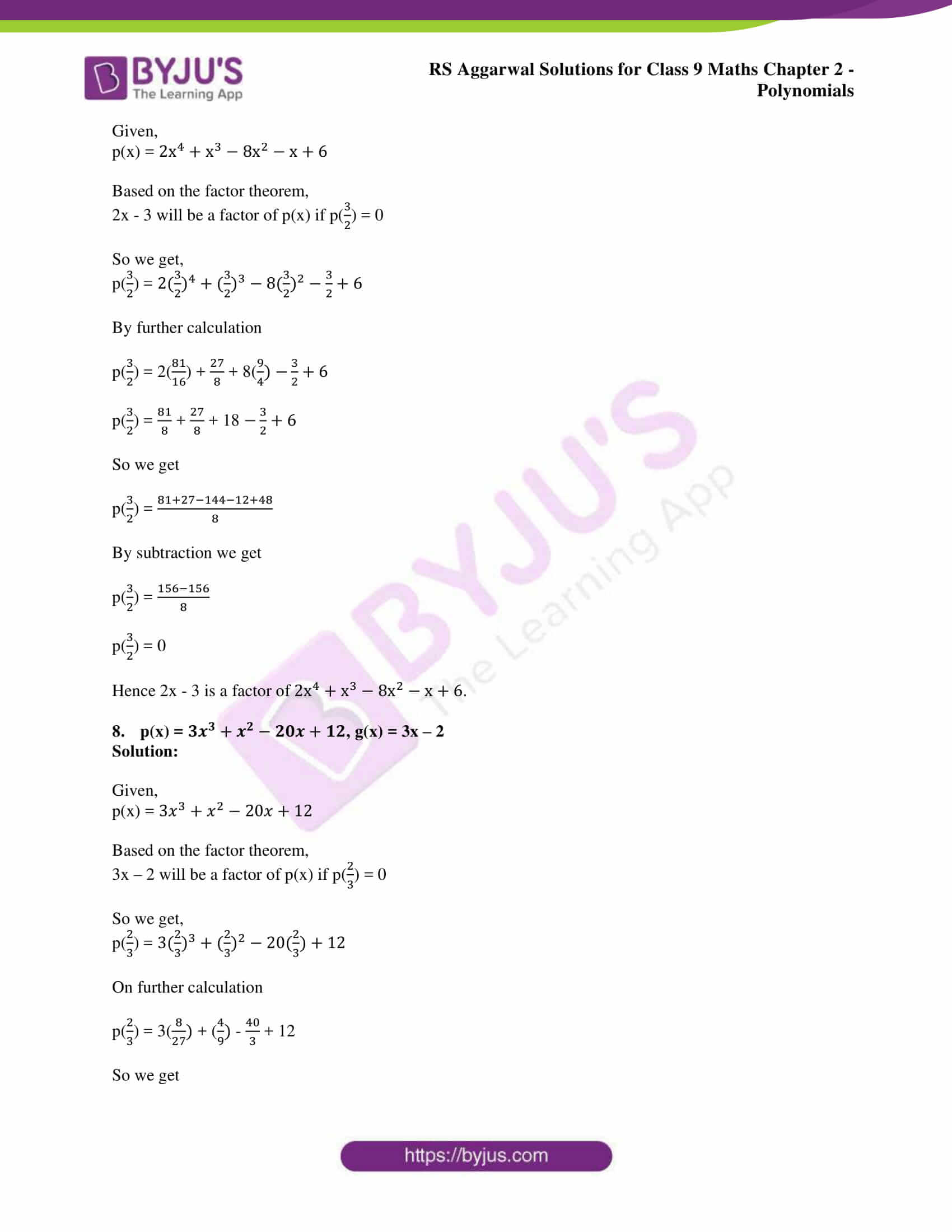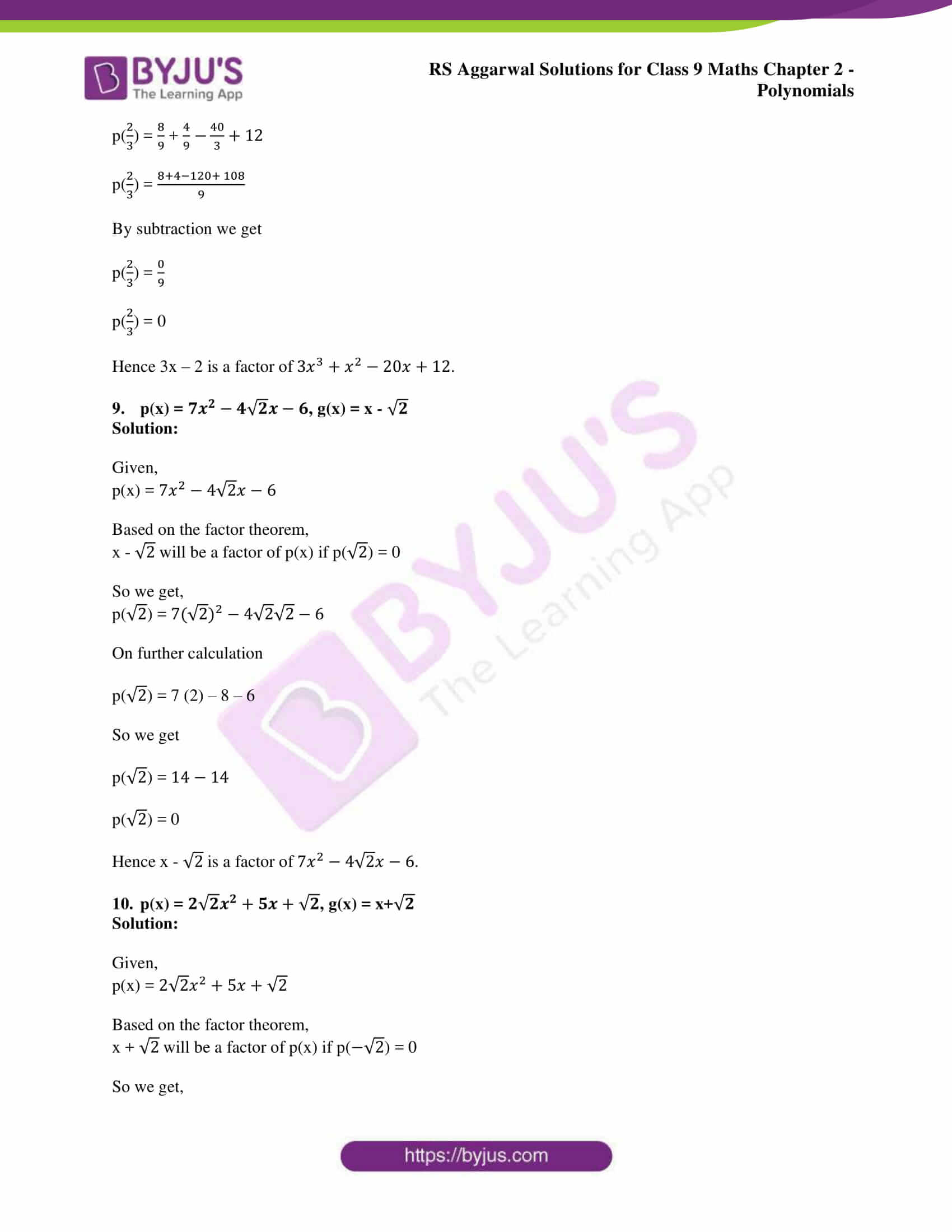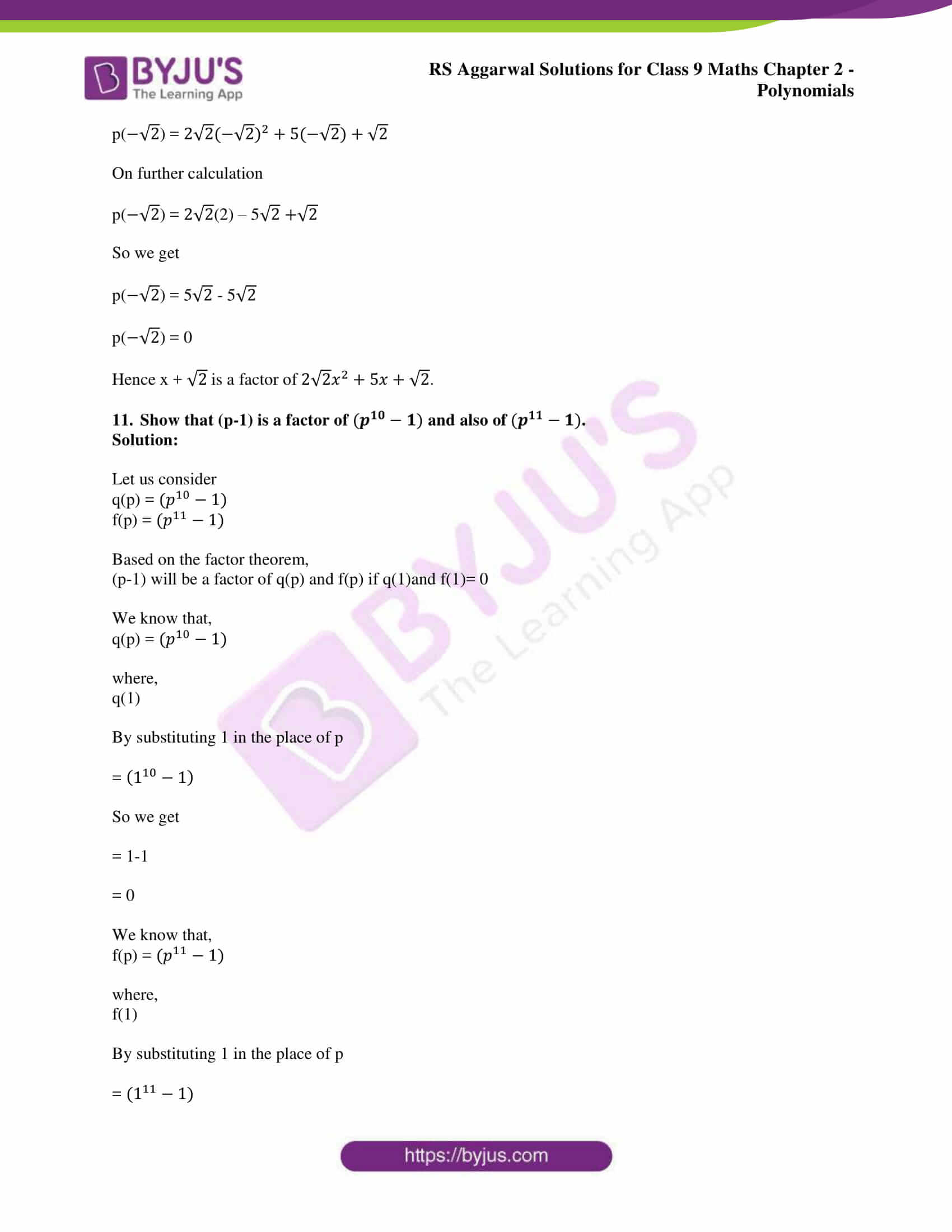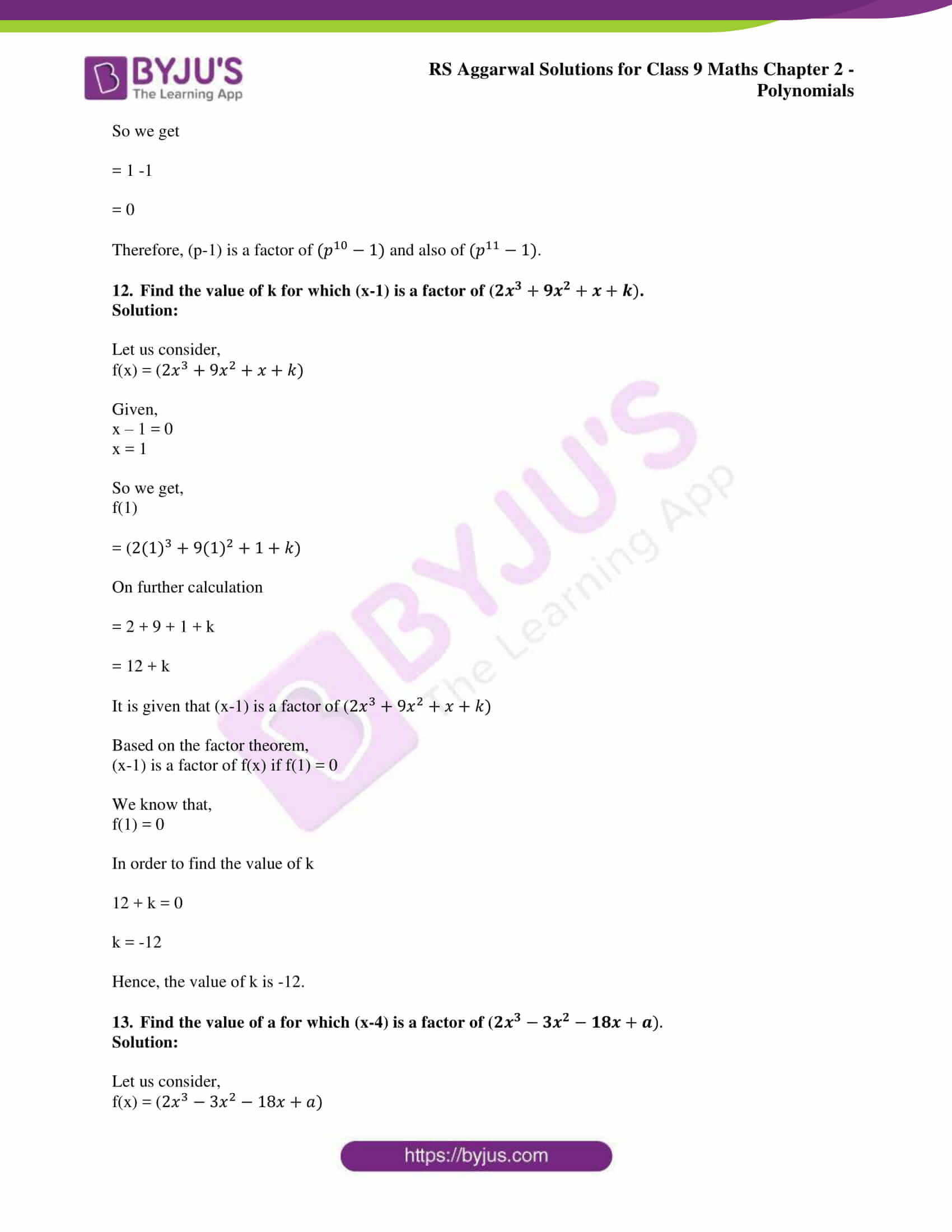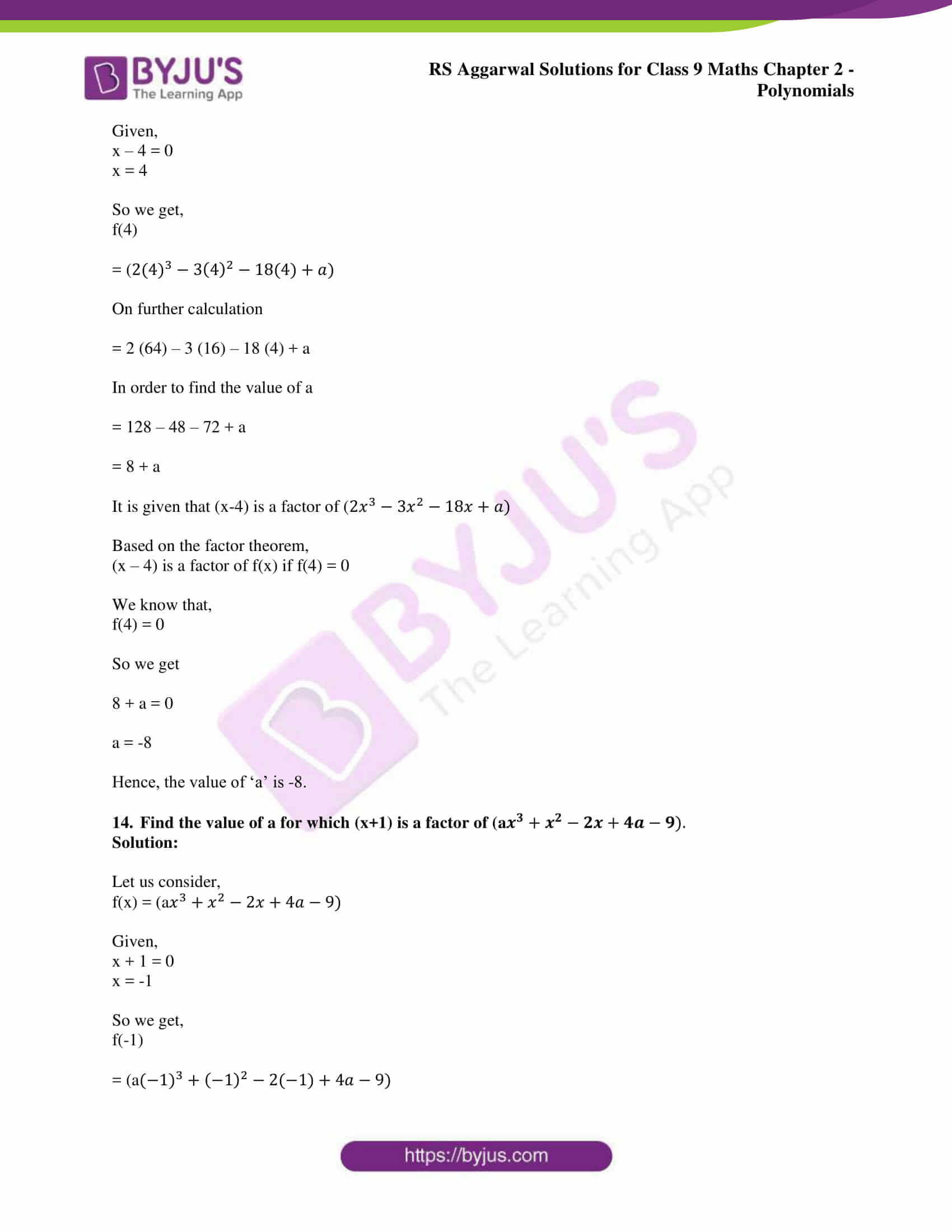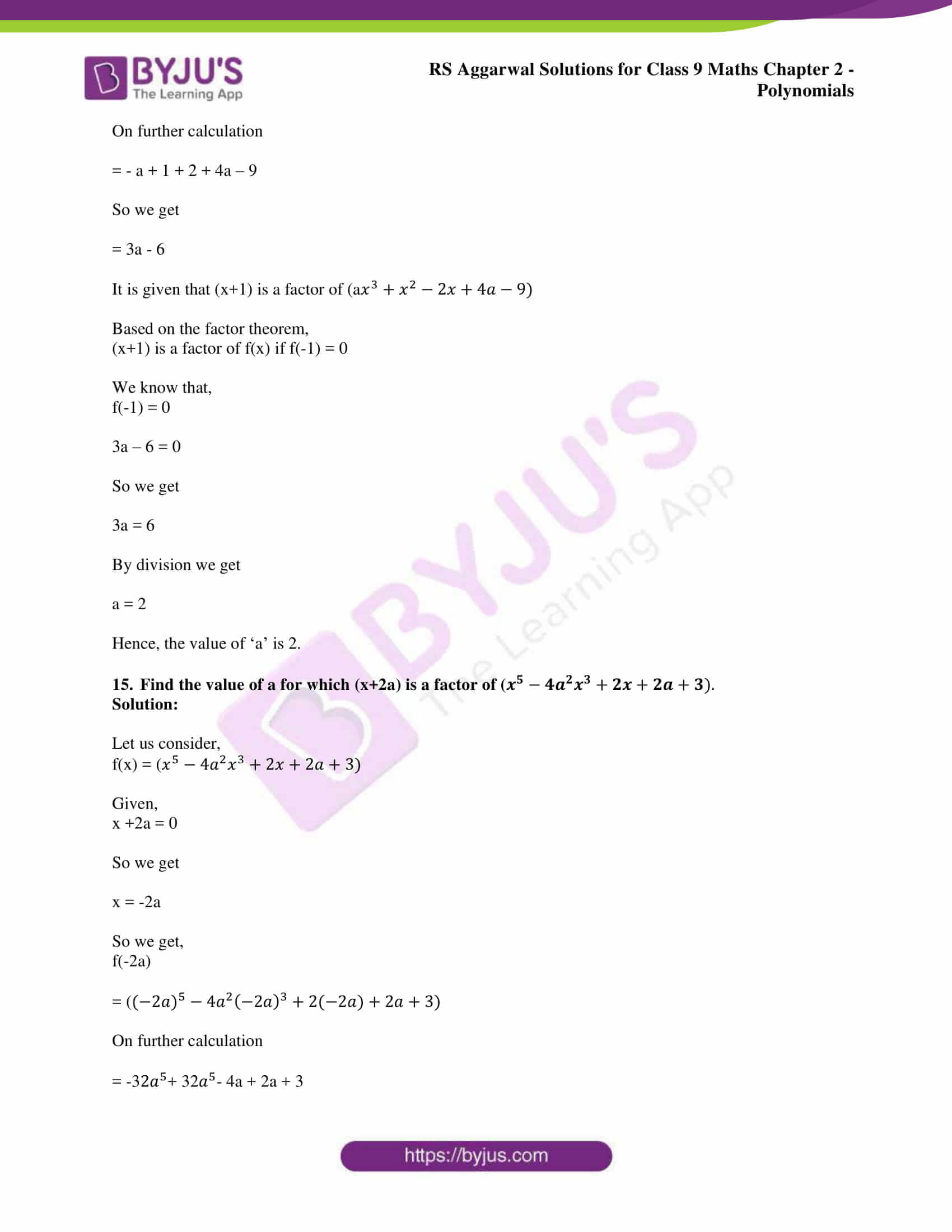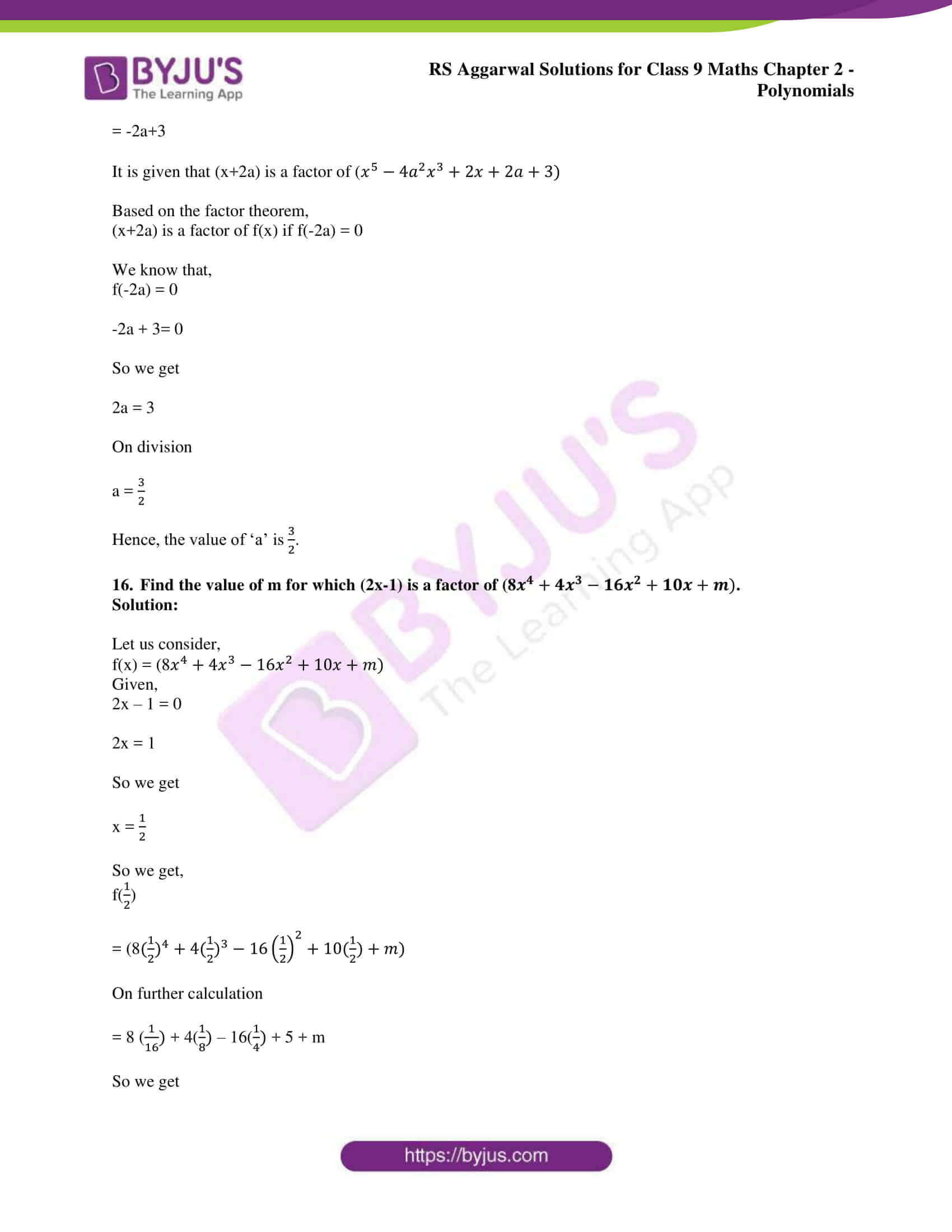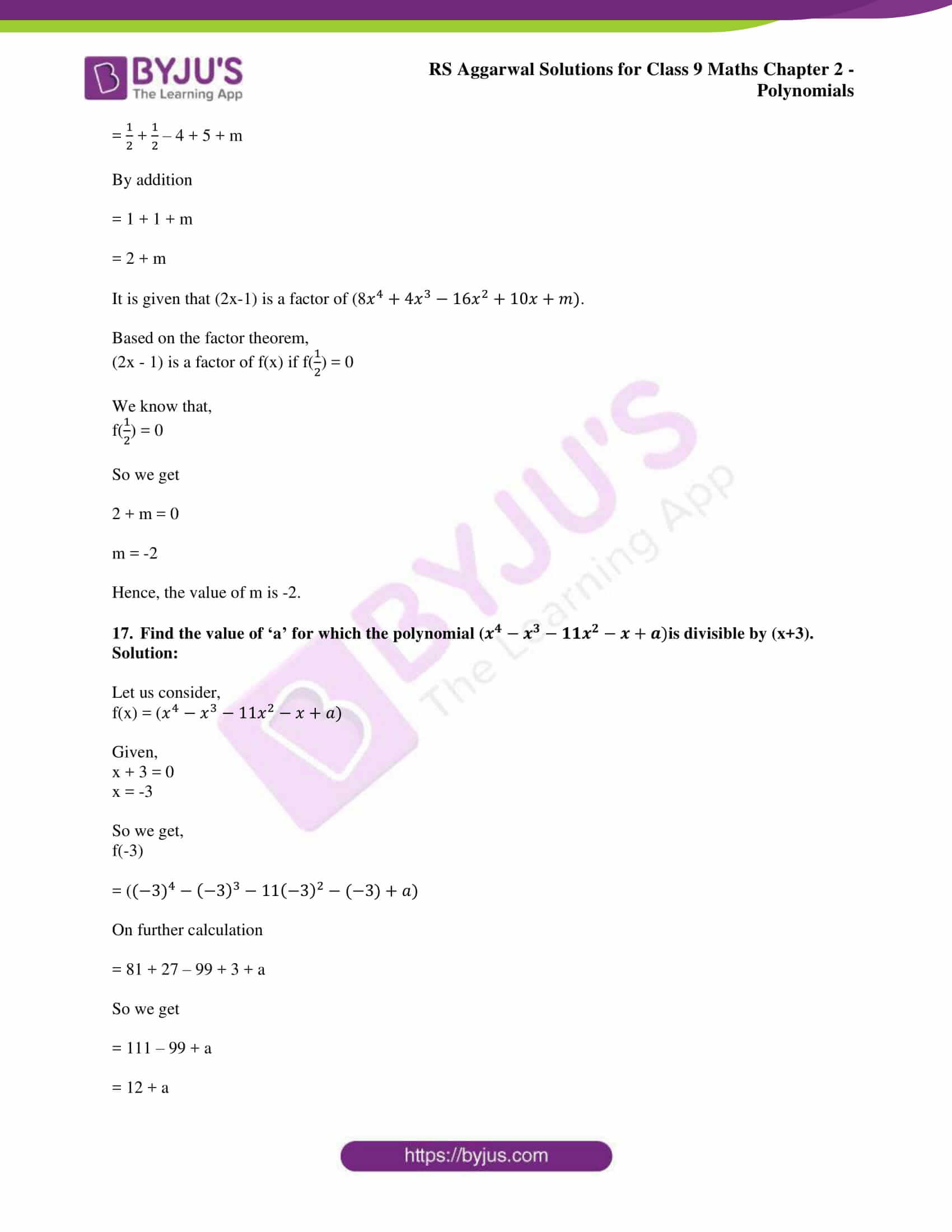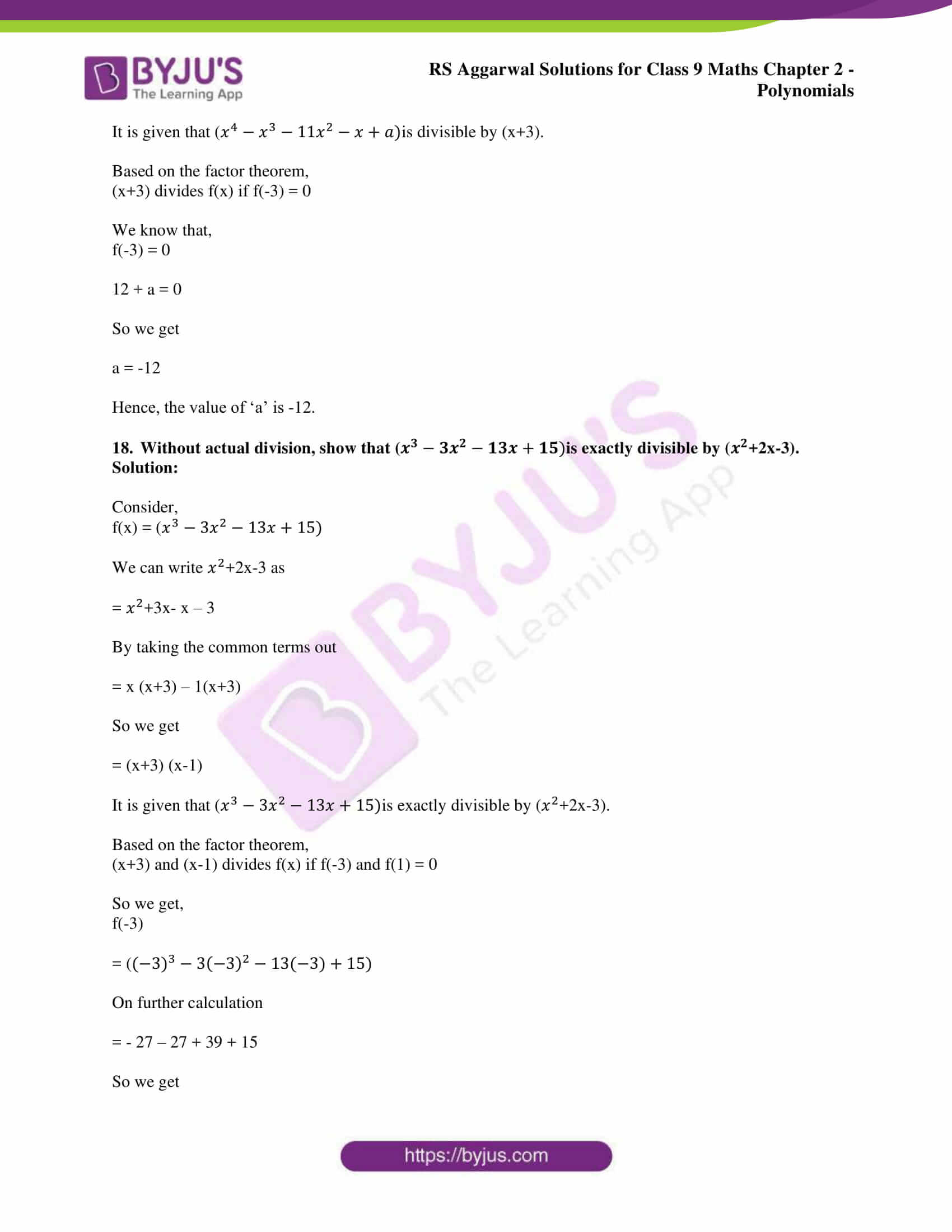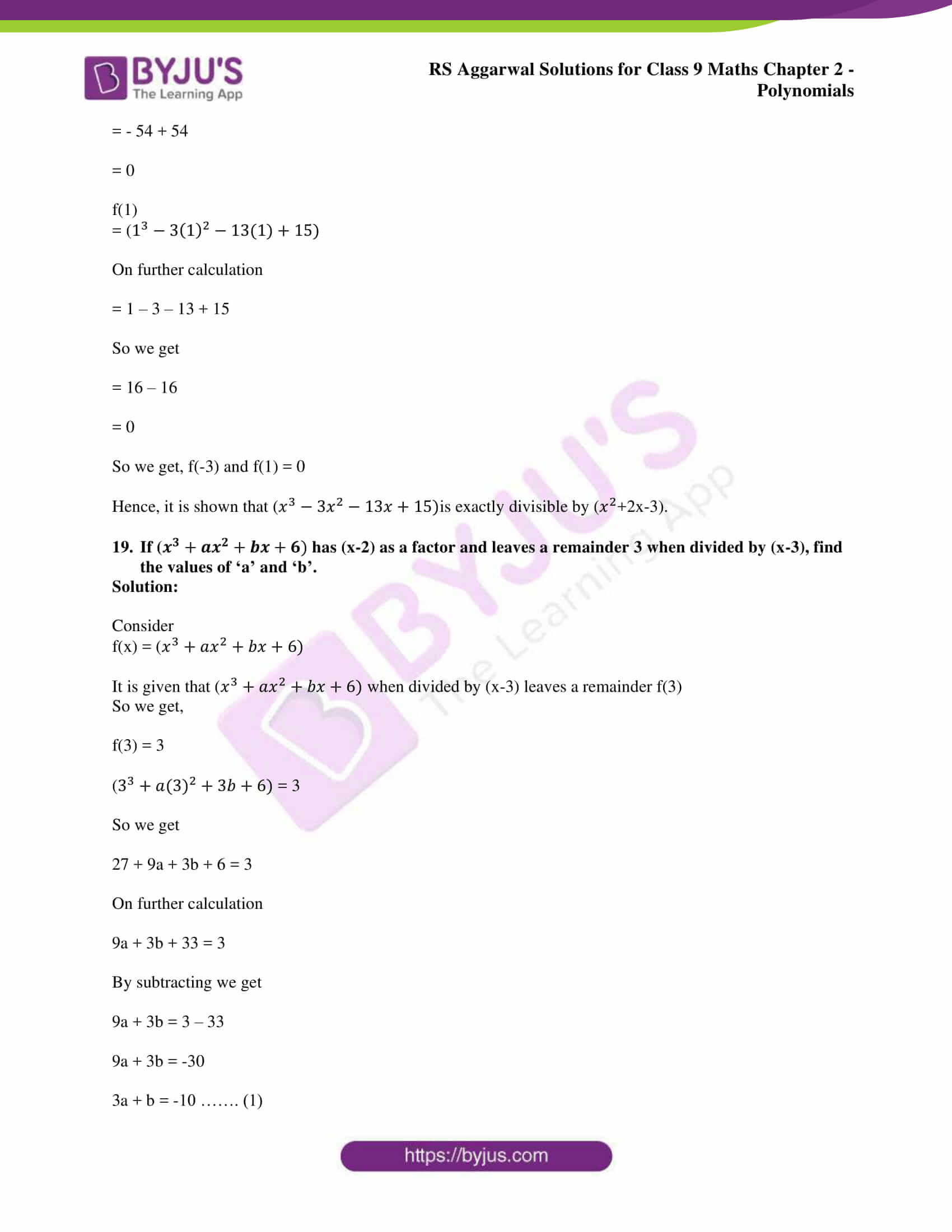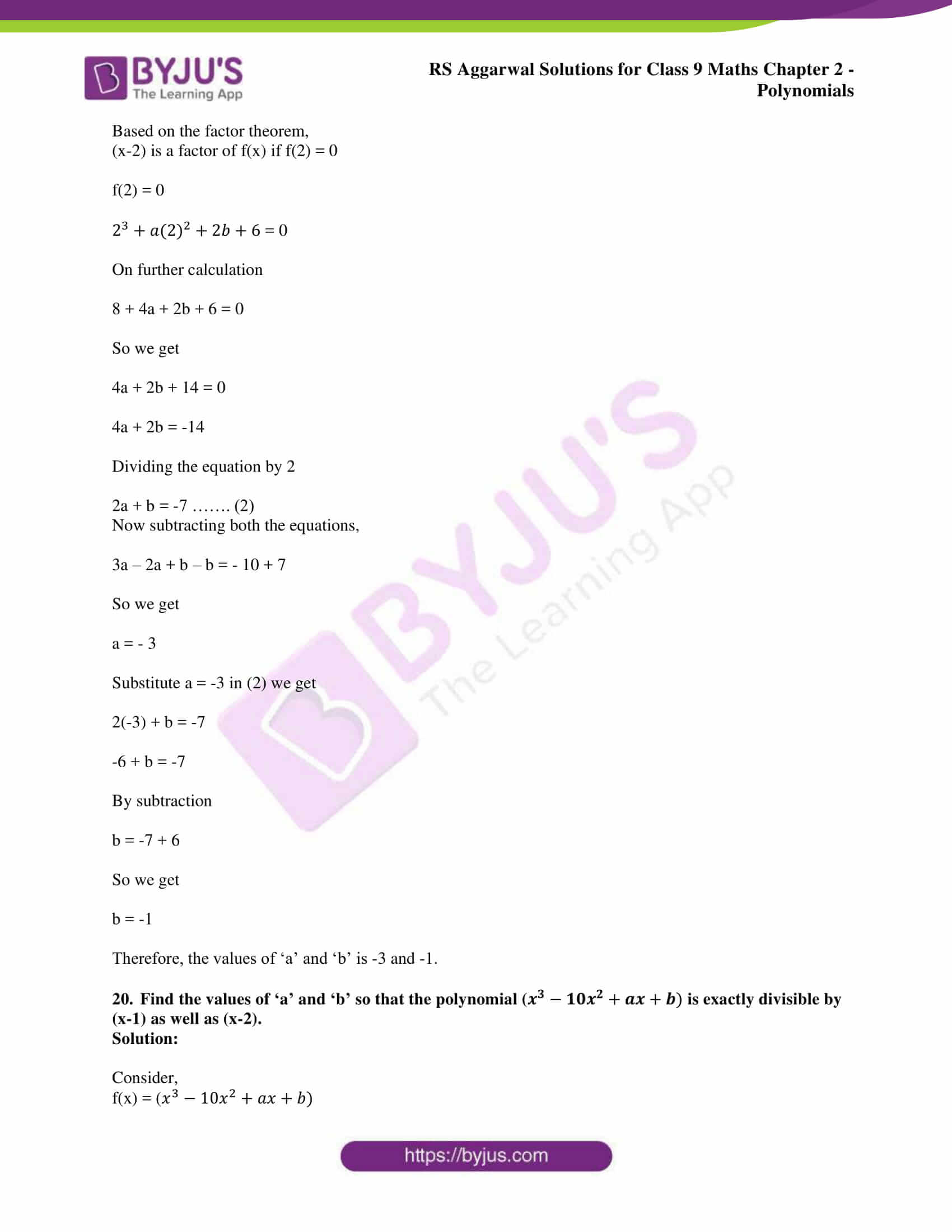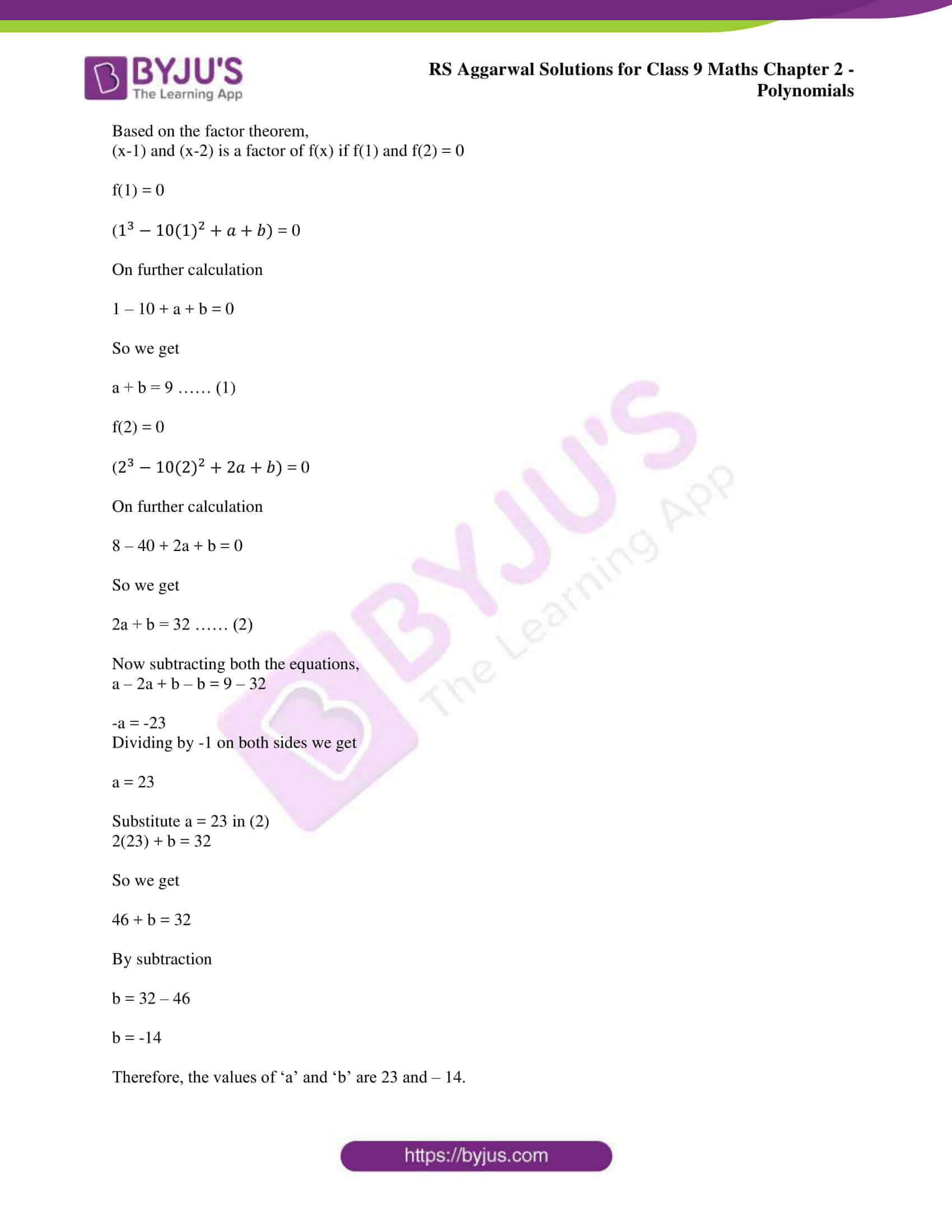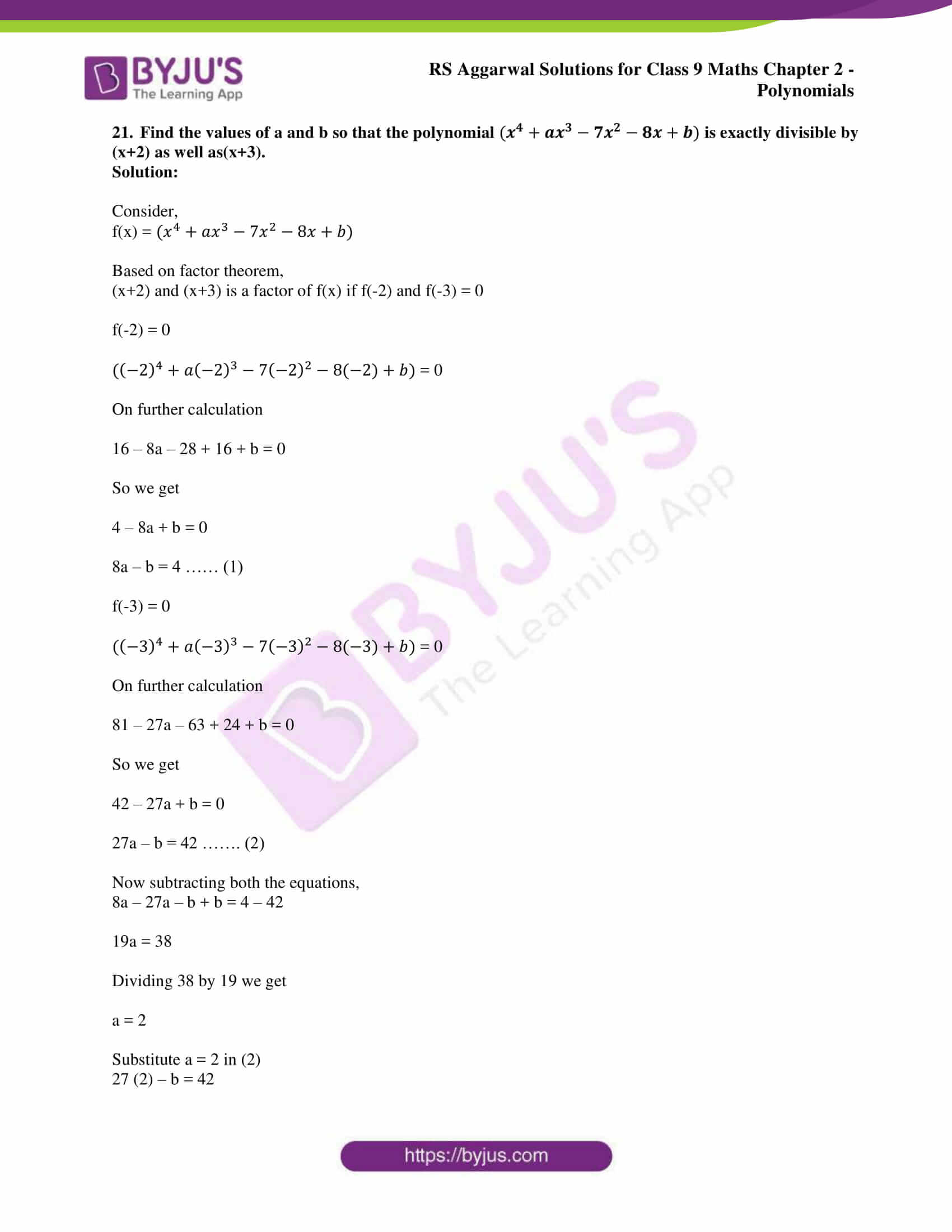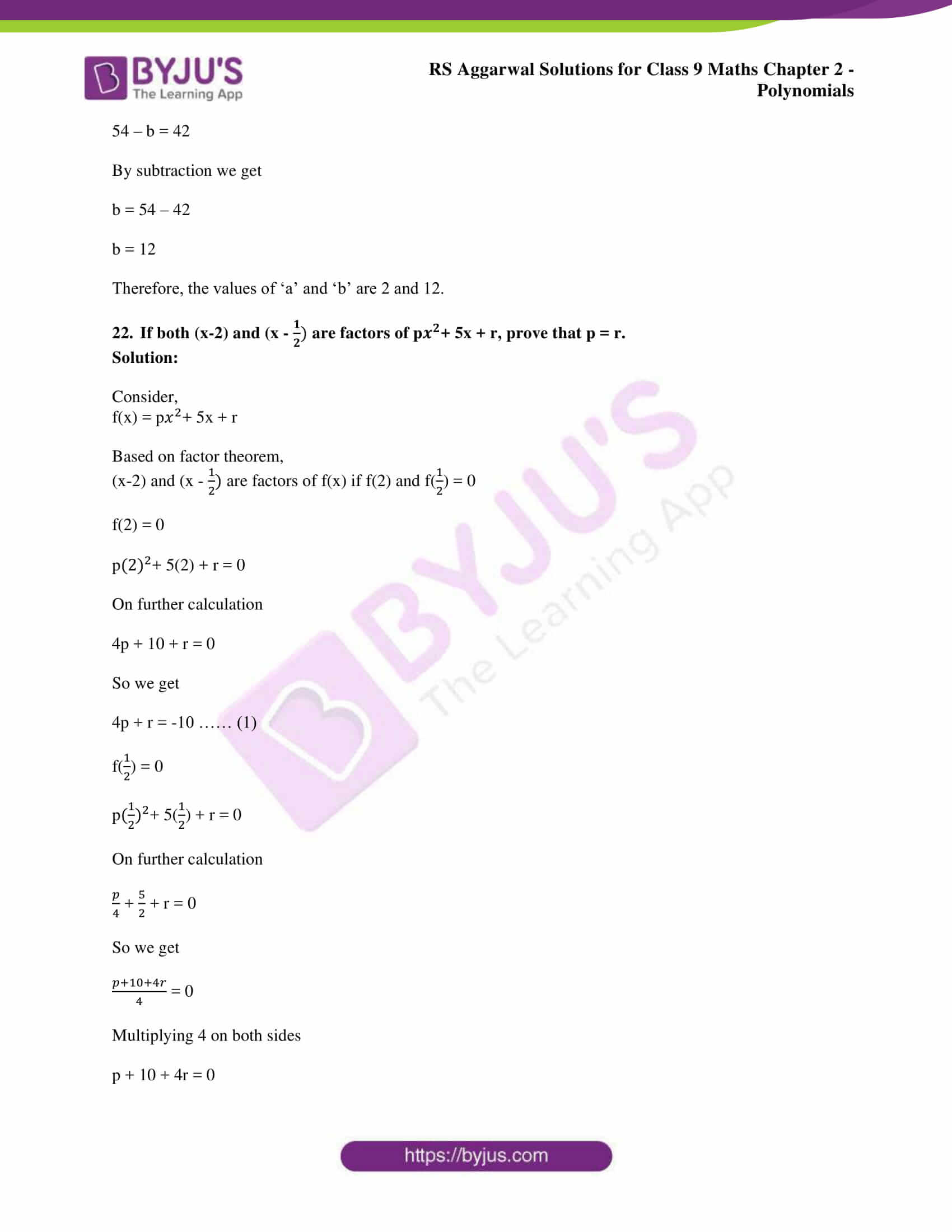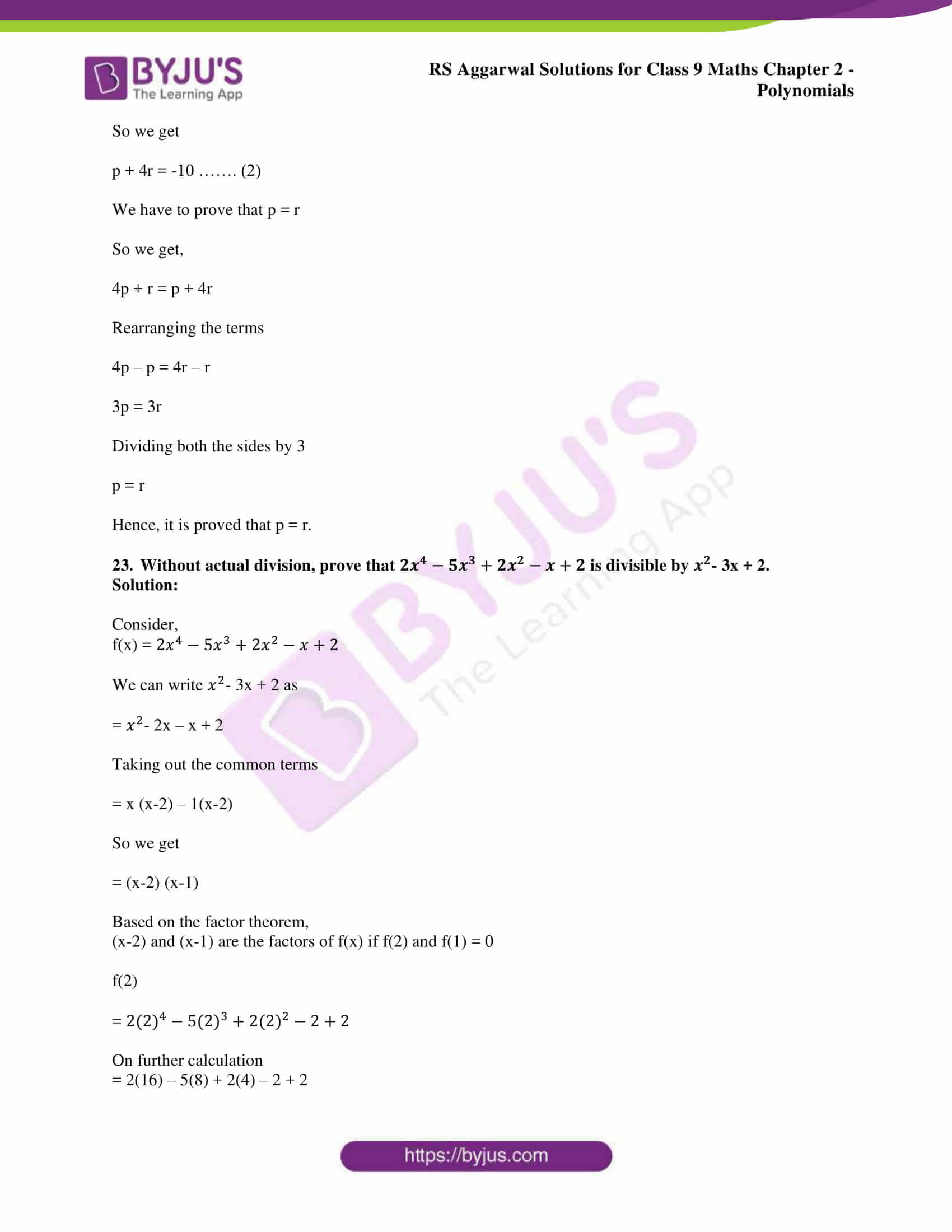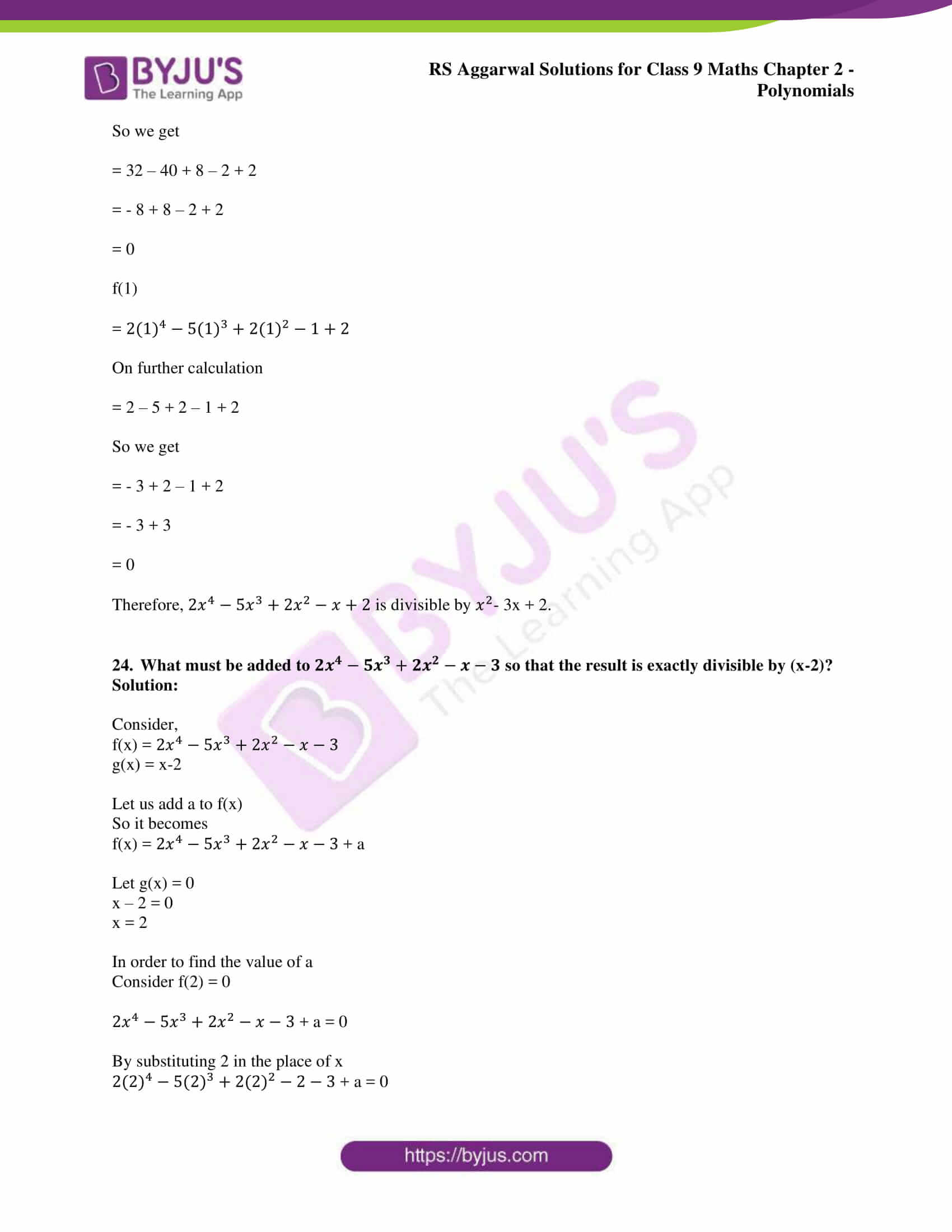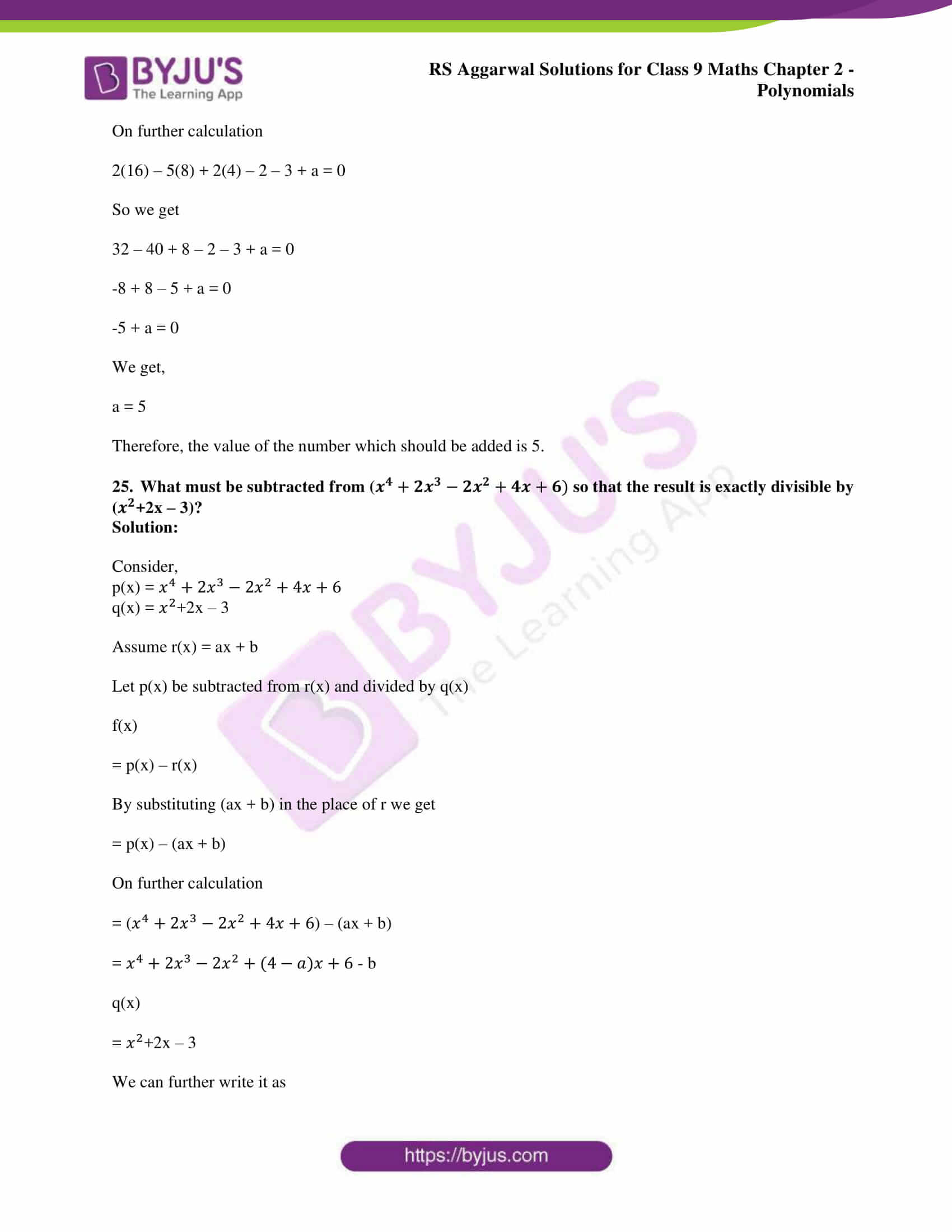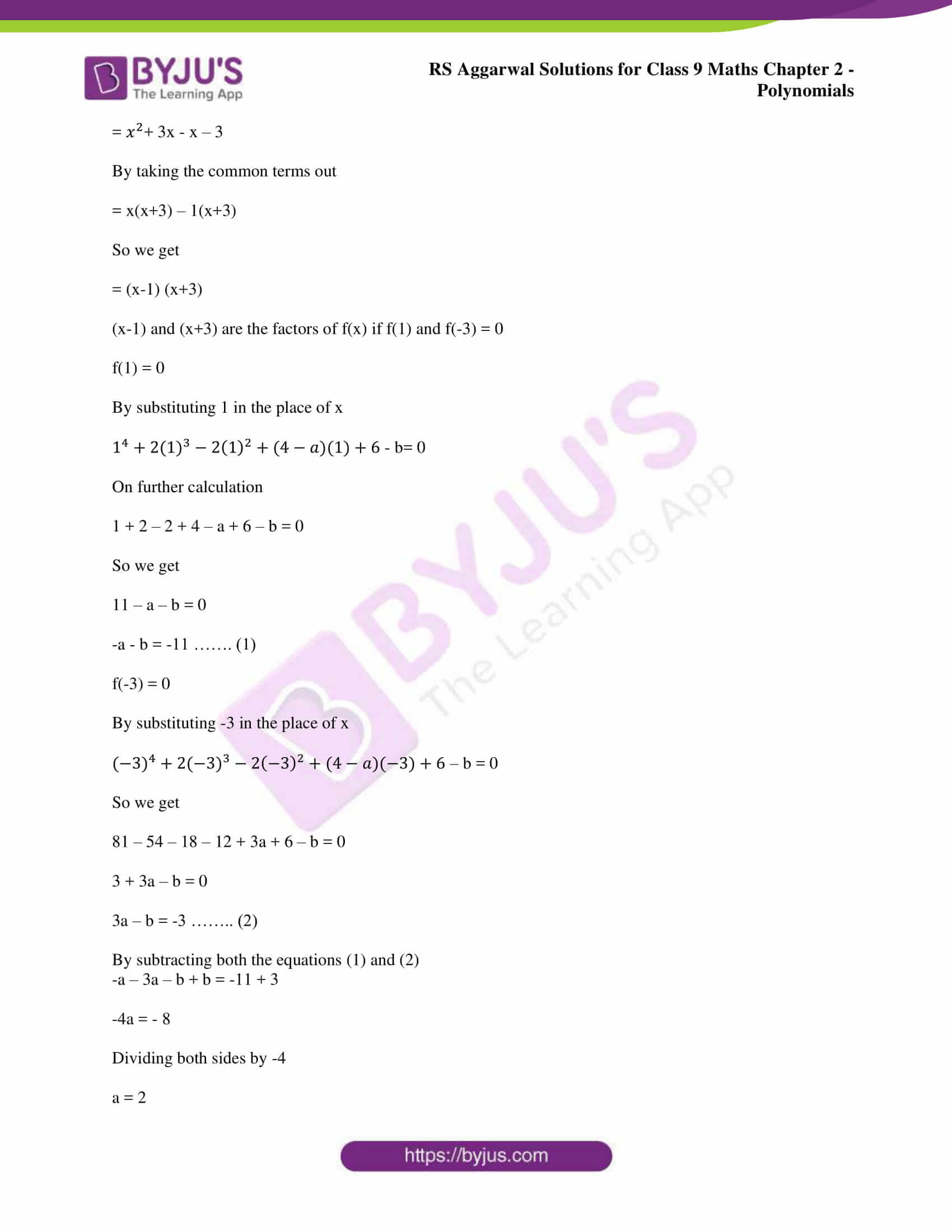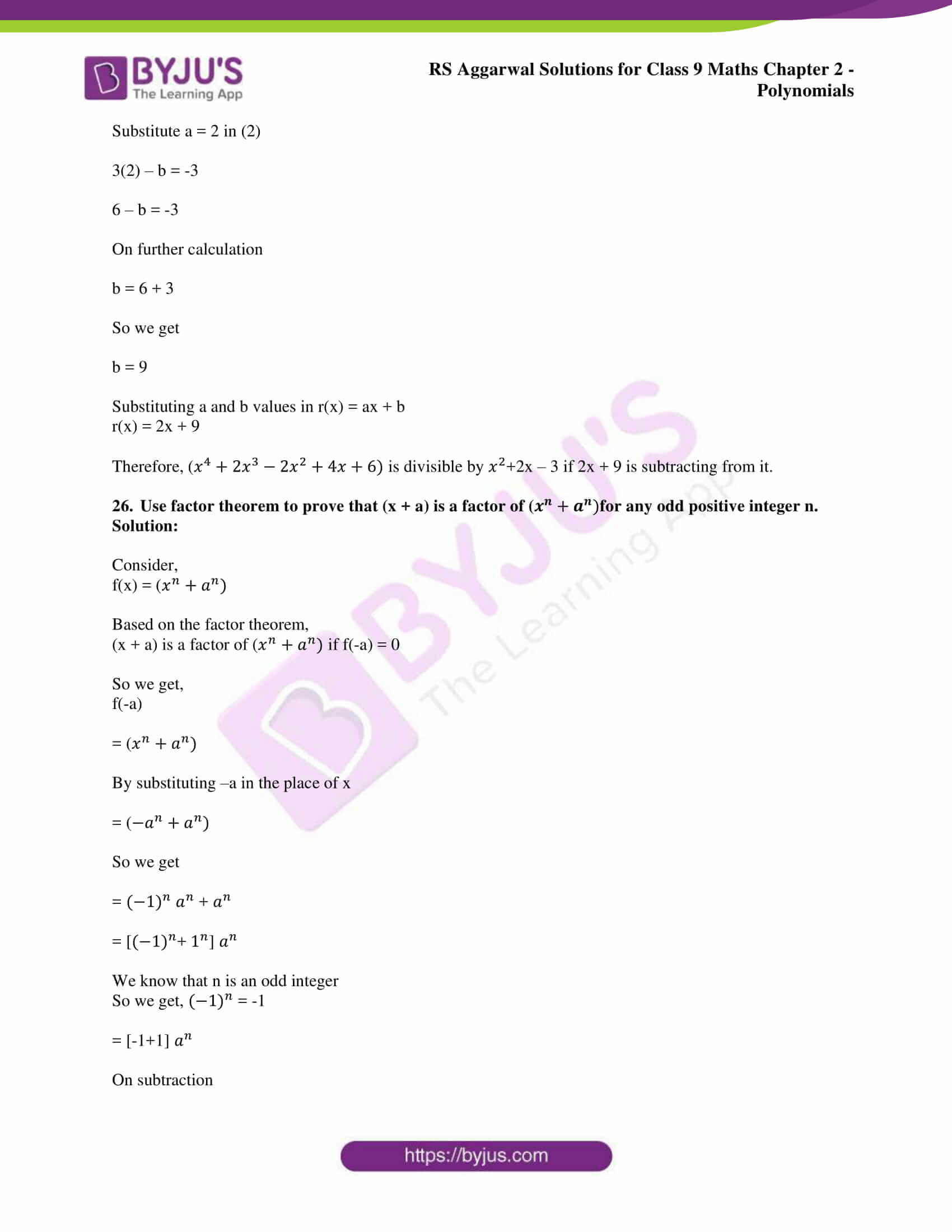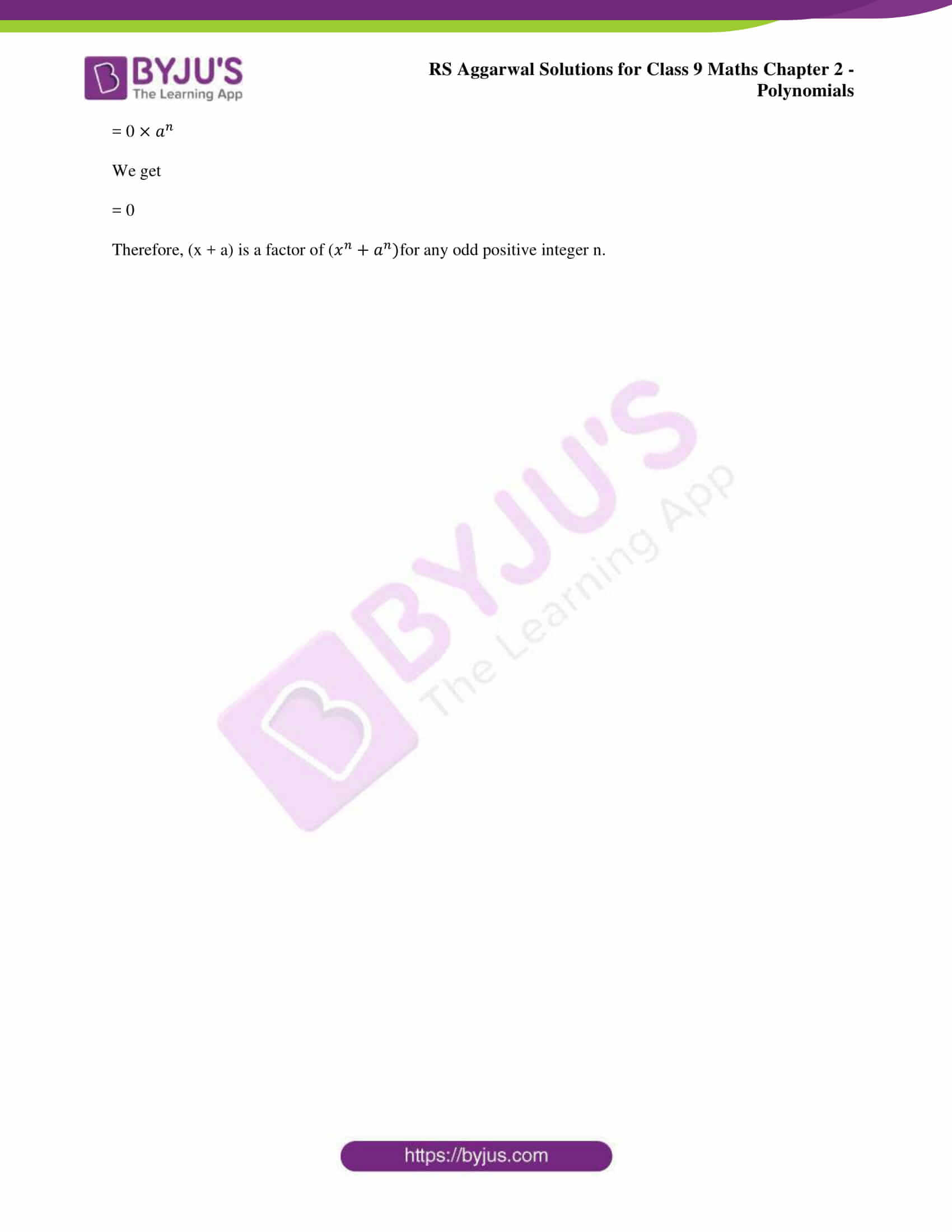### Access other exercise solutions of class 9 Maths Chapter 2: Polynomials

Exercise 2A Solutions 6 Questions

Exercise 2B Solutions 9 Questions

Exercise 2C Solutions 16 Questions

### RS Aggarwal Solutions Class 9 Maths Chapter 2 – Polynomials Exercise 2D

RS Aggarwal Solutions Class 9 Maths Chapter 2 Polynomials Exercise 2D mainly focuses on examples and problems which are solved using the Factor Theorem according to the current CBSE syllabus.

### Key features of RS Aggarwal Solutions for Class 9 Maths Chapter 2: Polynomials Exercise 2D

• The CBSE pattern followed in the RS Aggarwal textbook mainly helps students to understand the method of solving problems.
• Revising the syllabus by using the PDF of RS Aggarwal Solutions helps students to analyse topics they need to focus on.
• By solving many number of questions in each chapter helps students to manage their time effectively based on the exam duration.
• Exercise wise solutions can be referred by the students while solving the problems in order to gain more confidence in the concepts.